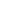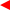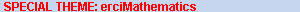contentsERCIM News No.50, July 2002# Quantitative Domain Theory

by Michel Schellekens

Domain Theory, a formal basis for the semantics of programming languages, originated in work by Dana Scott in the mid-1960s. Models for various types of programming languages, including imperative, functional, nondeterministic and probabilistic languages, have been extensively studied. Quantitative Domain Theory forms a new branch of Domain Theory and has undergone active research in the past three decades. The field involves both the semantics of programming languages and the mathematical field of Topology. Researchers at The National University of Ireland, Cork, focus on a recent development which has led to a partial solution of an open problem in Topology. Interestingly, the solution was guided by Computer Science intuitions.

Domain Theory originated in the revolutionary work by Strachey and Scott on the development of models (semantics) for programming languages. Scott’s work quickly led to connections with the mathematical fields of Topology and Category Theory. In this framework, the least fixed point of a functional, defined on a domain of Scott continuous functions and with range in the same set, is obtained as a topological limit. The topology involved is now known as the Scott topology.

An alternative to this essentially order theoretic approach was proposed by the Dutch school at CWI and originated in work by De Bakker, Zucker and America. This approach advocates the use of metrics.

In order to reconcile both approaches to Domain Theory, Smyth pioneered at Imperial College the use of methods from the field of Non-Symmetric Topology. This field traditionally studies ‘quasi-metrics’ which are obtained from classical metrics by removing the symmetry requirement. Hence, for quasi-metrics, the distance from a given point to a second point need not be the same as the converse distance. A simple example of a quasi-metric is the 0-1 encoding of a partial order which defines the distance between two points x and y to be 0 in case x is below y in the order and 1 otherwise.

Recent developments in Domain Theory indicate that additional concepts are required in order to develop the corresponding applications. These developments include domain theoretic approaches to dataflow networks, logic programming, domain theoretic approaches to integration, models for probabilistic languages as well as models which incorporate complexity analysis. An extensive series of papers has been published in this last area, by the author in collaboration with Salvador Romaguera (Polytechnical University of Valencia). Each of these applications involve ‘real number measurements’ in some sense, and hence the adjective ‘quantitative’ is used as opposed to the adjective ‘qualitative’ which indicates the traditional order theoretic approach.

The question remains as to how ‘quantities’ can be introduced to classical Domain Theory in a simple and elegant way. Even though we favour weightable quasi-metric spaces, introduced by Matthews at Warwick, our approach is to view Quantitative Domain Theory in first instance as an extension of traditional Domain Theory via a minimal fundamental concept: that of a semivaluation. A semivaluation is a novel mathematical notion which generalizes the fruitful notion of a valuation on a lattice to the context of semilattices.

The semivaluation approach has the advantage that it allows for a uniform presentation of the traditional quantitative domain theoretic structures and applications, as for instance the totally bounded Scott domains of Smyth and the partial metric spaces of Matthews.

A topological problem stated by the Swiss Mathematician Hans-Peter Kunzi essentially required the mathematical characterization of partial metrics. Interestingly, such a characterization has been obtained based on the notion of a semivaluation. This notion was directly motivated by computer science examples. Hence the result forms an example of recent developments where the mathematical area of Topology is influenced by Computer Science. Traditionally the influence has largely been in the opposite direction.

The benefit to Computer Science is that semivaluations allow for the introduction of a suitable notion of a ‘quantitative domain’ which can serve to develop models for the above mentioned applications. This work has been reported in two recent papers, both of which will appear in the Elsevier journal ‘Theoretical Computer Science’, and which are available from our webpage: ‘The correspondence between partial metrics and semivaluations’ and its sequel: ‘A characterization of partial metrizability. Domains are quantifiable.’

Current investigations in this area are under way at UCC as part of a Boole Centre for Research in Informatics BCRI-project led by the author, in collaboration with Maria O’Keeffe and, via Enterprise Ireland and Royal Irish Academy projects in collaboration with researchers from the University of Siegen, the University of Birmingham and Imperial College London.